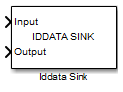# Iddata Sink

Export simulation data as `iddata` object to MATLAB workspace

• Library:
• System Identification Toolbox

•## Description

The Iddata Sink block exports simulation data as an `iddata` object to the MATLAB® workspace. The object stores the input and simulated output signals, sampled at the sample time that you specify. If you simulate your model from the model window, the block exports the object to the MATLAB base workspace. If you simulate the model programmatically, the object is exported to the MATLAB caller workspace. The caller workspace is the workspace of the function that called the currently running function.

## Ports

### Input

expand all

Input of `iddata` object, specified as a scalar for single-input data. For multichannel data with Nu inputs, specify `Input` as a vector of length Nu.

Data Types: `double`

Output of `iddata` object, specified as a scalar for single-output data. For multichannel data with Ny outputs, specify `Output` as a vector of length Ny.

Data Types: `double`

## Parameters

expand all

Name of `iddata` object, specified as a MATLAB variable name. The object is exported with this name to the MATLAB workspace.

Sample time in seconds, specified as a finite positive number. The `iddata` object stores the input and output signals, sampled at the sample time that you specify.

## Version History

Introduced in R2008a